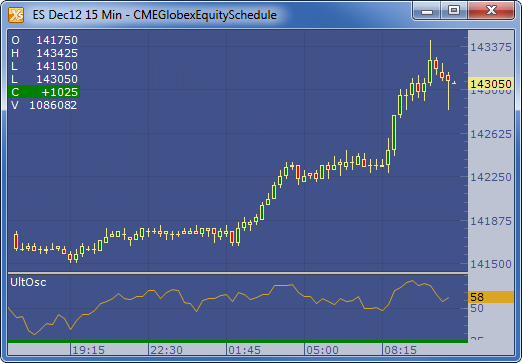← Back to X_TRADER® Help Library

### X_STUDY® Documentation

#### Ultimate Oscillator (ULTOSC)

##### Description

The Ultimate Oscillator (ULTOSC) by Larry Williams is a momentum oscillator that incorporates three different time periods to improve the overbought and oversold signals.

##### Formula

ULTOSC = 100 x [(4 x Average7)+(2 x Average14)+Average28]/(4+2+1)

Where:

Average7 = (7-period BP Sum) / (7-period TR Sum)

Average14 = (14-period BP Sum) / (14-period TR Sum)

Average28 = (28-period BP Sum) / (28-period TR Sum)

BP = Buying Power = Close - Minimum(Low or Previous Close).

TR = True Range = Maximum(High or Previous Close) - Minimum(Low or Previous Close)

##### Example